# Mathematics Paper 1 Questions and Answers - Form 3 End Term 3 2022 Exams

## QUESTIONS

SECTION 1 (50 Marks)
Answer ALL questions from this section

1. Evaluate: (2 Marks)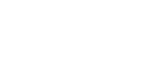2. Solve the equation. (4 Marks)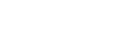3. The first, third and seventh terms of an increasing arithmetic progression are the three consecutive terms of a geometric progression. If the first term of the arithmetic progression is 10, find the common difference of the arithmetic progression. (3 Marks)
4. Find y if log2y – 2 = log292 (3 Marks)
5. Rationalize the denominator (3 Marks)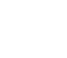6. A contractor employs 40 men to do a piece of work in 60 days each man working 9 hours a day. The contractor is then required to do the same job in 48 days. How many more men working 10 hours a day does he need to employ. (3 Marks)
7. A student’s results in six Mathematics tests were: 24, 28, 32, x, 48 and 50. If the median is 36, find the mean mark. (3 Marks)
8. Given that the dimensions of a rectangle are 20.0cm and 25.0cm. Find the percentage error in calculating the area. (3 Marks)
9. The co-ordinates of the points P and Q are (1,-2) and (4,10) respectively. A point T divides the line PQ in the ratio 2:1
Determine the co-ordinates of T (2 Marks)
10. A student at a certain college has a 60% chance of passing an examination at the first attempt. Each time a student fails and repeats the examination his chance of passing is increased by 15%.
Calculate the probability that a student in the college passes an examination at the second or at the third attempt. (4 Marks)
11. What is the exact value of: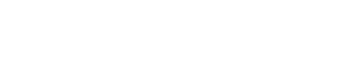(2 Marks)
12. A salesman earns a basic wage of KSh. 1500 per week in addition, he is paid commission per week as follows:-
Commission
For sales upto KSh. 50,000                                0%
For sales above KSh. 50,000
1. For the first KSh. 25,000                               2%
2. For the next KSh. 25000                             2½%
3. For any amount above KSh.100,000           5%
During that week, he sold goods worth KSh. 115,000. What was his total pay for that week. (4 Marks)
13. Two grades of tea A and B costs KSh. 25 and KSh. 28 respectively per kg. They are mixed and the mixtures sold at KSh. 31.20 making a profit of 20%. Find the ratio of A:B in the mixture. (4 Marks)
14. The surface area of two similar bottles are 12cm2 and 108cm2 respectively. If the bigger one has a volume of 810cm3. Find the volume of the smaller one. (3 Marks)
15. If tan x = 1/√3, Find without tables or calculator,
Sin(90-x) + Cos (90 –x). Leave your answer in surd form in simplest form. (3 Marks)
16. A regular polygon has the sum of all its interior angles as 1260º. Find the size of each exterior angles in the polygon. (3 Marks)

SECTION II

1. A group of people planned to contribute equally towards a water project which needed KSh. 2,000,000 to complete. However, 40 members of the group withdrew from the project. As a result each of the remaining members were to contribute KSh. 2500 more.
1. Find the original number of members in the group. (5 Marks)
2. 45% of the value of the project was funded by Consistuency Development Fund (CDF). Calculate the amount that would be made by each of the remaining members of the group. (3 Marks)
3. Members contribution were in terms of labour provided and money contributed. The ratio of the value of labour to the money contributed was 6:19, calculate the total amount of money contributed by members. (2 Marks)
2.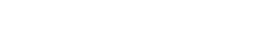1. 2a + 3b (2 Marks)
2. ½a – b (2 Marks)
3. If x and y are scalars in the following equation.
xa – yb =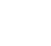, form two equations simultaneously hence solve for x and y. (6 Marks)
3. The figure below shows two intersecting circles of centres C and D radii 16cm and 20cm respectively. The two circles substend angles θ1 and θ2 at their centres respectively and intersect at P and Q as shown.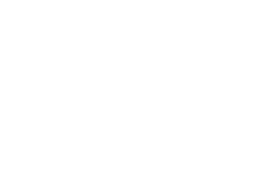Given that the area of triangle PCQ = 80.14cm2,
Calculate the size of
1. The angle marked θ1 (2 Marks)
2. The angle marked θ2 (3 Marks)
3. The area of the shaded region (5 Marks)
4. The diagram below represents a solid frustrum consisting of a hemispherical bottom and a conical frustrum at the top.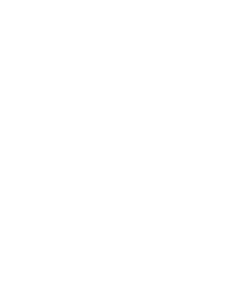1. Calculate the value of x( height of the smaller one) (2 Marks)
2. Calculate:
1. Surface area of the solid (4 Marks)
2. Volume of the solid (4 Marks)
5. Town B is 102km on a bearing of 112º from town A. Town C is 94 km on a bearing of 062º from town B. Town D is 073º from town A and 336º from town C.
1. Using a scale of 1cm rep. 20km, draw a diagram to show the positions of towns A, B, C and D.
2. Using the diagram in (a) above, determine
1. Bearing of town B from town D. (1 Mark)
2. Bearing of town A from town C (1 Mark)
3. The distance AC and BD (2 Marks)
6. A surveyor recorded the measurements of a field in a field book using lines AB 260m as shown below.
 B 130 R    40 70 Q    10 50 P     20 S50 10 A
1. Sketch the map of the field (4 Marks)
2. Find the area of the field in hectares (6 Marks)
7. Construct the parallelogram ABCD where AB = 8cm, BC = 6cm angle ABC = 1200. Using a ruler and a pair of compass only. (3 Marks)
1. Draw in the diagram diagonals and construct the circumcircle ABD (2 Marks)
2. Drop a perpendicular from D to meet AB. Let the perpendicular cut diagonal AC at x. (2 Marks)
3. Drop a perpendicular from B to meet DC and cut diagonal AC at Y. (2 Marks)
4. Measure XY (2 Marks)
8.
1. Complete the table below for y = 2x3 + x2 – 5x + 2.
For the interval -3≤x≤3. (2 marks)
 x -3 -2 -1 0 0.5 1 2 3 2x3 -54 -2 0.25 16 x2 9 4 0.25 1 -5x 5 0 -2.5 -5 -10 +2 2 2 2 2 2 2 2 2 y 6 50
2. Draw the graph of y = 2x3 + x2 – 5x + 2 for the interval -3≤x≤3 (3 Marks)
3. Use your graph to solve equation y = 2x3 + x2 – 5x + 2 (1 Mark)
4. Use your graph to solve equation y = 2x3 + x2 – 11x – 10 (2 Marks)
5. Find the gradient of the curve at x = 2 (2 Marks)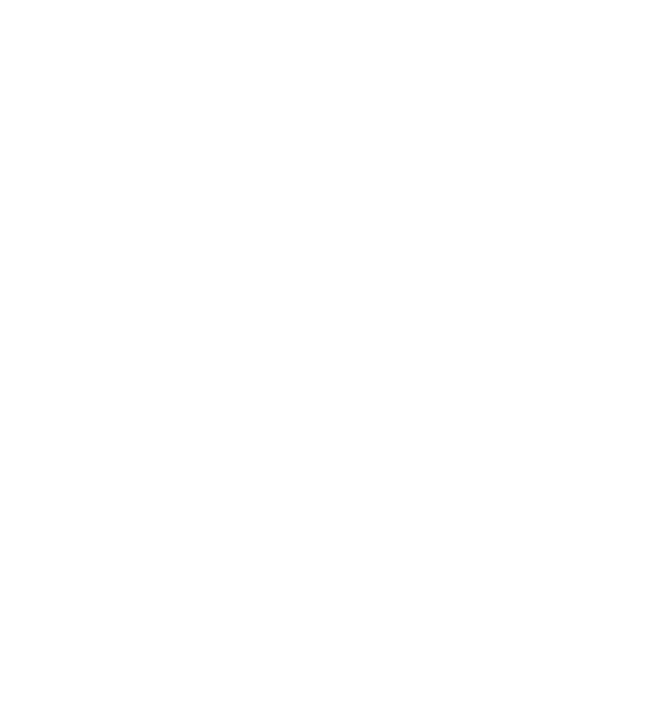## MARKING SCHEME

No.

1.

Numerator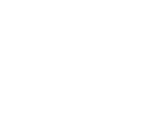Denominator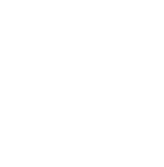2.

L.C.M. 10x
x  + 1 x 10x - 3/x10x = x - 2x 10x
2                                5
5x(x + 1) – 30 = 2x(x – 2)
5x2 + 5x – 30 = 2x2 – 4x
3x2 + x – 30 = 0
3x2 + 10x – 9x – 30 = 0 (10,-9)
x(3x + 10)-3(3x + 10) = 0
(x – 3) (3x + 10) = 0
x – 3 = 0 = 3
3x + 10 = 0 = -10
3

3.

T1 = 10 + 0d = 10
T3 = 10 + 2d
TT = 10 + 6d
10 + 2d = 10 + 6d
10         10 + 2d
(10 + 2d)(10 + 2d) = 10(10 + 6d)
100 + 40d + 4d2 = 100 + 60d
60d = 40d + d2
4d2 = 20d
d = 5

4 Log2y 2 = Log292
Log2y – Log292 = 2
Log2 (y/92)= 2
y/92 = 22
y = 4 x 92
y = 368

5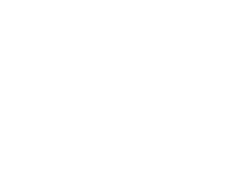6

Men    Days     Hours
40       60          9
?         48          10
No. of men to work 48 days 10 hrs
40 x 60 x 9
48 x 10
= 45
= 45 – 40 = 5 Men

7

Mean – of six
n , n + 1
2   2
32, x
Median 36 = 32 + x
2
72 = 32 + x
72 - 32 = x
40 = x

Mean mark
= 24 + 28 + 32 + 40 + 48 + 50
6
x = 37

8

Absolute error
= max - working + working - min
2
=20.05 x 25.05 - 19.95 x 24.95
2
= 4.5
2
= 2.25
% error =  absolute x 100
actual
=        2.25     x 100
20.0 x 25.0
= 0.45%

9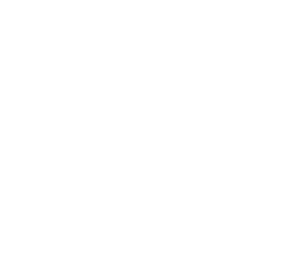10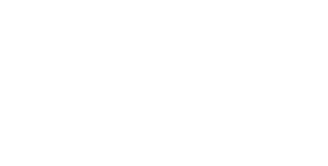P (Second or third)
= P(FP) or P(FFP)
= 0.4 x 0.69 + 0.4 x 0.31 x 0.7935
= 0.276 + 0.098394
= 0.374394

11 2w(x - 2)2
y + 1
X = 3

Y = 3 + 3 = 6
W = 2 x 3 + 6
= 6 + 6 = 12
2 x 12(3 - 2)2
6 + 1
24 x 12 = 24
7        7
x = 3, y = x + 3 and w = 2x + y

12

The 1st 25000 = 2500 x 2/100= 500/=
Next 25000 = 25000 x  2.5/100 = 625/=
Reamaining 15,000
= 15000 x 5/100 = 750/=
Total commission = 500 + 625 + 750
= 1875/=
Weekly pay 1500 + 1875 = 3375/=

13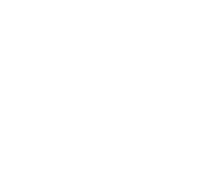14

A.S.F = 108:12 = 9:1
L:S:F = √A.S.F or A.S.F = (L.S.F)2
L:S:F = 3 :1
(L:S:F)3 = V.S.F
V.S.F = 27:1
Volume of smaller = 1/27 x 810
= 30cm3

15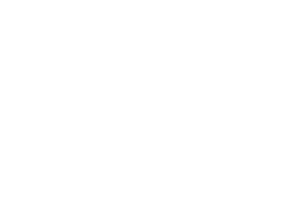16

Sum = (2n – 4)90 = 1260
2n – 4 = 14
= 2n = 18
2       2
n = 9 (No. of sides)
Size of exteruior = 360
n
= 400

17
1. Let the group members be x
Originally, each member was to contribute
2,000,000
x
After withdrawal of 40 members, each member had to contribute.
2,000,000
x -40
But, the same amount was to be raised despite the number of members.
Therefore:
2,000,000 = 2,000,000  – 2500
x             x - 40
(x-4) 2,000,000 = 2,000,000  x (x-4) – x(x-4)(2500)
x                x - 40
2,000,000 x – 8,000,000 = 2,000,000x – 2500x2 + 100000x
25x2 – 1000x – 800,000 = 0
x2 – 40x – 32,000 = 0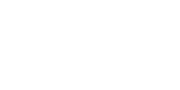X = 200 or – 160
Original no. of members = 200
2. Fund from CDF =  45  x  2,000,000 = 900,000
100
Amount remaining to be contributed
2,000,000 – 900,000 = 1,100,000
Amount contributed by each member =
= 1,100,000 =  6875/=
160

18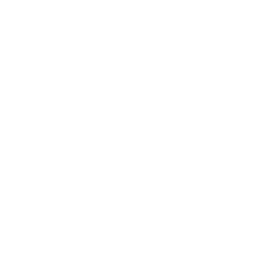c). Given xa – yb = (-13)
(-22)
Form a simultaneous equation hence find x and y (6 Marks)
Using substitution and elimination.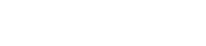1. 3x – 14y = -13
2. -4x – y = -22
from (i) 3x = -13 + 14y
x = 13 + 14y
3
-4(-13 + 14y) - y = -22
3
52-56y – 3y = -66
-59y = -118
y = 2
3x – 14y = -13
3x – 14 x 2 = -13
3x = -13 + 28
3x = 15
x = 5

19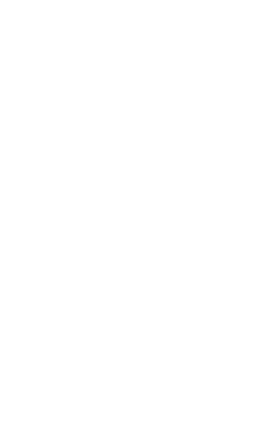Sin 19.38 =    PX
16 cm
PX = 16sin 19.380
= 5.3cm3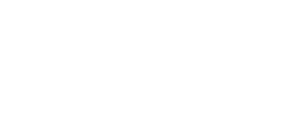Sin θ2 = 5.3cm
20cm
θ2 = 15.37 x 2
= 30.730

20
1.    x       2.1
x + 4       4.9
4.9x = 2.1x + 8.4
x = 3cm
Height = 3cm +4cm = 7cm
2. A = πr2 = (22 x 4.9 x 4.9) x 2
7
= 150.92cm2
Area of frustrum
Slant  √72 + 4.92 = 8.545cm (Big cone)
=  √2.12 + 32 = 3.662cm (Small cone)
=( 22  x 49 x 8.545)–( 22  x 2.1 x 3.662)
7                              7
131.593 – 24.1692
107.4238cm2

Total surface area
= 150.92 + 107.4238
= 258.34cm2
volume of hemisphere
2 x 22  x 4.93 = 246.5 cm
3    7
Volume of frustrum:
x 22 x 4.92 x 7) – ( 1 x 22  x 2.12 x 3)
3      7                         3     7
176.07 – 13.86
= 162.21cm3

21
1.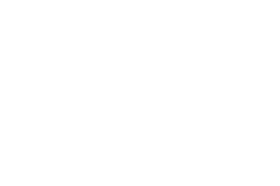2. Bearing of town B from town D
= 2120 ± 10
Bearing of town A from C
= 2680±10

3. Distance
AC = 9cm x 20km = 180km
BD = 5.4cm x 20km = 108km

22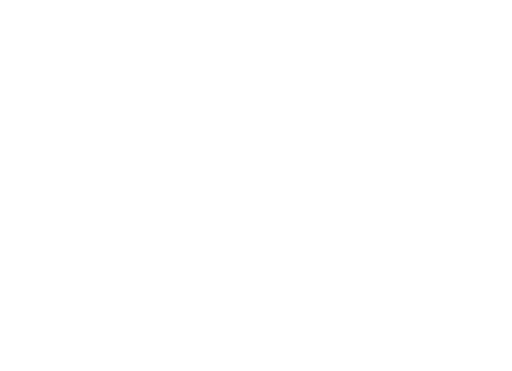23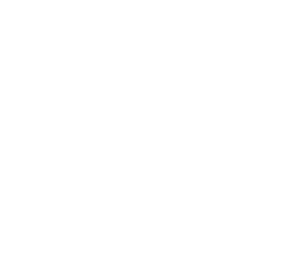24(a)
 x -3 -2 -1 0 0.5 1 2 3 2x3 -54 -16 -2 0 0.25 1 16 54 x2 9 4 1 0 0.25 1 4 9 -5x 15 10 5 0 -2.5 -5 -10 +2 2 2 2 2 2 2 2 2 y -28 0 6 2 0 0 12 50

B2 If all values
B1 Any 4 values of y

24(b)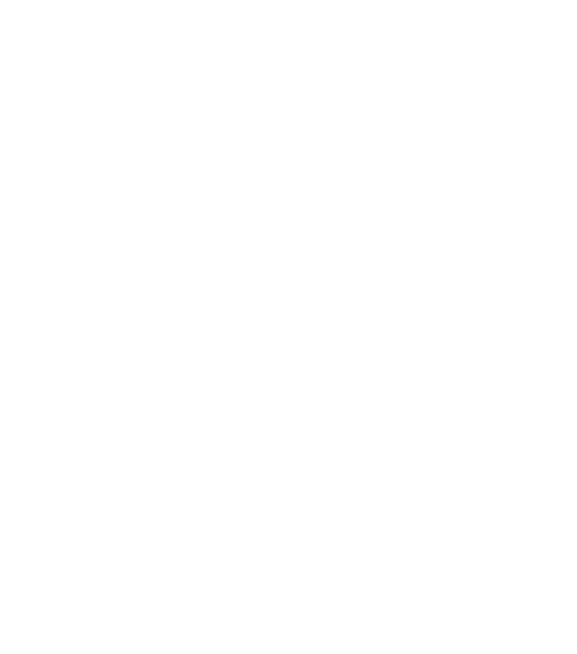24(c) - (e)

(c) = (-2,0.5,1)±0.1
(d) Line y = 6x + 12
x = -2,-1,2.5±0.1
(e)    0 - 2.0   = 1.667
1.3 - 2.5

• ✔ To read offline at any time.
• ✔ To Print at your convenience
• ✔ Share Easily with Friends / Students